College Algebra with Corequisite Support 2e

# 6.2Graphs of Exponential Functions

College Algebra with Corequisite Support 2e6.2 Graphs of Exponential Functions

### Learning Objectives

• Graph exponential functions.
• Graph exponential functions using transformations.

### Corequisite Skills

#### Learning Objectives

• Graph exponential functions (IA 10.2.1).
• Function transformations (exponential) (CA 3.5.1-3.5.5).

### Example 1

Graph exponential functions.

On the same coordinate system graph $f(x)=2xf(x)=2x$ and $g(x)=2x+1.g(x)=2x+1.$

On the same coordinate system graph $f(x)=3xf(x)=3x$ and $g(x)=3x−2.g(x)=3x−2.$

##### Practice Makes Perfect
1.

On the same coordinate system graph $f(x)=3xf(x)=3x$ and $g(x)=3x-1.g(x)=3x-1.$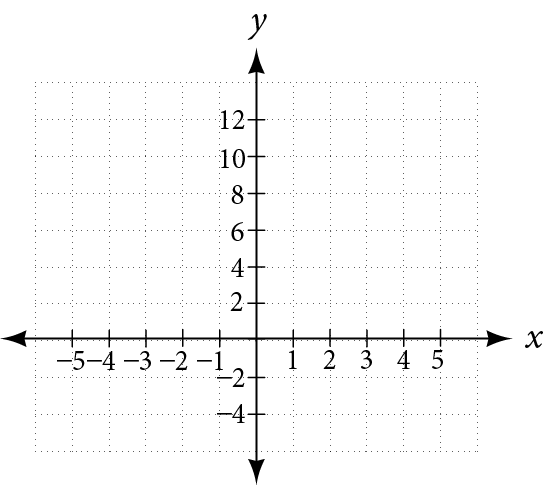2.

On the same coordinate system graph $f(x)=3xf(x)=3x$ and $g(x)=3x+1.g(x)=3x+1.$#### Objective 2: Function transformations (exponential). (CA 3.5.1-3.5.5)

Vertical and Horizontal Shifts: Given a function $f(x)f(x)$ , a new function $g(x)=f(x)+kg(x)=f(x)+k$ where $kk$ is a constant, is a vertical shift of the function $f(x)f(x)$. All the output values change by k units. If k is a positive, the graph will shift up. If k is negative, the graph will shift down.

Given a function $f(x)f(x)$ , a new function $g(x)=f(x-h)g(x)=f(x-h)$ , where h is a constant, is a horizontal shift of the function $f(x)f(x)$ . If h is positive, the graph will shift right. If h is negative, the graph will shift left.

### How To

Given a function and both a vertical and a horizontal shift, sketch the graph.

1. Step 1. Identify the vertical and horizontal shifts from the formula.
2. Step 2. The vertical shift results from a constant added to the output. Move the graph up for a positive constant and down for a negative constant.
3. Step 3. The horizontal shift results from a constant added to the input. Move the graph left for a positive constant and right for a negative constant.
4. Step 4. Note the order of the shifts, transformations, and reflections follow the order of operations.

### Example 2

Function transformations (exponential).

Graph $f(x)=3x+2-3f(x)=3x+2-3$

### How To

Given a function, reflect the graph both vertically and horizontally.

1. Multiply all outputs by –1 for a vertical reflection. The new graph is a reflection of the original graph about the x-axis.
2. Multiply all inputs by –1 for a horizontal reflection. The new graph is a reflection of the original graph about the y-axis.
##### Practice Makes Perfect

Function transformations (exponential).

3.

Graph $f(x)=2x-3-1f(x)=2x-3-1$4.
• Given $f(x)=3xf(x)=3x$ , reflect it about y-axis and write an equation of a new function below.
• Given $f(x)=3xf(x)=3x$ , reflect it about x-axis and write an equation of a new function below.
• Given $f(x)=3xf(x)=3x$ , shift the graph up 4 units and write an equation of a new function below.
• Graph the equations found in parts a, b, and c on the coordinate system provided and check your work using a graphing utility.

As we discussed in the previous section, exponential functions are used for many real-world applications such as finance, forensics, computer science, and most of the life sciences. Working with an equation that describes a real-world situation gives us a method for making predictions. Most of the time, however, the equation itself is not enough. We learn a lot about things by seeing their pictorial representations, and that is exactly why graphing exponential equations is a powerful tool. It gives us another layer of insight for predicting future events.

### Graphing Exponential Functions

Before we begin graphing, it is helpful to review the behavior of exponential growth. Recall the table of values for a function of the form $f(x)= b x f(x)= b x$ whose base is greater than one. We’ll use the function $f(x)= 2 x . f(x)= 2 x .$ Observe how the output values in Table 1 change as the input increases by $1. 1.$

 $x x$ $−3 −3$ $−2 −2$ $−1 −1$ $0 0$ $1 1$ $2 2$ $3 3$ $f(x)= 2 x f(x)= 2 x$ $1 8 1 8$ $1 4 1 4$ $1 2 1 2$ $1 1$ $2 2$ $4 4$ $8 8$
Table 1

Each output value is the product of the previous output and the base, $2. 2.$ We call the base $2 2$ the constant ratio. In fact, for any exponential function with the form $f(x)=a b x , f(x)=a b x ,$ $b b$ is the constant ratio of the function. This means that as the input increases by 1, the output value will be the product of the base and the previous output, regardless of the value of $a. a.$

Notice from the table that

• the output values are positive for all values of $x; x;$
• as $x x$ increases, the output values increase without bound; and
• as $x x$ decreases, the output values grow smaller, approaching zero.

Figure 1 shows the exponential growth function $f(x)= 2 x . f(x)= 2 x .$

Figure 1 Notice that the graph gets close to the x-axis, but never touches it.

The domain of $f(x)= 2 x f(x)= 2 x$ is all real numbers, the range is $( 0,∞ ), ( 0,∞ ),$ and the horizontal asymptote is $y=0. y=0.$

To get a sense of the behavior of exponential decay, we can create a table of values for a function of the form $f(x)= b x f(x)= b x$ whose base is between zero and one. We’ll use the function $g(x)= ( 1 2 ) x . g(x)= ( 1 2 ) x .$ Observe how the output values in Table 2 change as the input increases by $1. 1.$

 $x x$ $-3-3$ $-2-2$ $-1-1$ $00$ $11$ $22$ $33$ $g ( x ) = ( 1 2 ) x g ( x ) =( 1 2 ) x$ $88$ $44$ $22$ $11$ $1 2 1 2$ $1 4 1 4$ $1 8 1 8$
Table 2

Again, because the input is increasing by 1, each output value is the product of the previous output and the base, or constant ratio $1 2 . 1 2 .$

Notice from the table that

• the output values are positive for all values of $x; x;$
• as $x x$ increases, the output values grow smaller, approaching zero; and
• as $x x$ decreases, the output values grow without bound.

Figure 2 shows the exponential decay function, $g(x)= ( 1 2 ) x . g(x)= ( 1 2 ) x .$

Figure 2

The domain of $g(x)= ( 1 2 ) x g(x)= ( 1 2 ) x$ is all real numbers, the range is $( 0,∞ ), ( 0,∞ ),$ and the horizontal asymptote is $y=0. y=0.$

### Characteristics of the Graph of the Parent Function $f(x)= b x f(x)= b x$

An exponential function with the form $f(x)= b x , f(x)= b x ,$ $b>0, b>0,$ $b≠1, b≠1,$ has these characteristics:

• one-to-one function
• horizontal asymptote: $y=0 y=0$
• domain:
• range: $(0,∞) (0,∞)$
• x-intercept: none
• y-intercept: $( 0,1 ) ( 0,1 )$
• increasing if $b>1 b>1$
• decreasing if $b<1 b<1$

Figure 3 compares the graphs of exponential growth and decay functions.

Figure 3

### How To

Given an exponential function of the form $f(x)= b x , f(x)= b x ,$ graph the function.

1. Create a table of points.
2. Plot at least $3 3$ point from the table, including the y-intercept $( 0,1 ). ( 0,1 ).$
3. Draw a smooth curve through the points.
4. State the domain, $( −∞,∞ ), ( −∞,∞ ),$ the range, $( 0,∞ ), ( 0,∞ ),$ and the horizontal asymptote, $y=0. y=0.$

### Example 1

#### Sketching the Graph of an Exponential Function of the Form f(x) = bx

Sketch a graph of $f(x)= 0.25 x . f(x)= 0.25 x .$ State the domain, range, and asymptote.

### Try It #1

Sketch the graph of $f(x)= 4 x . f(x)= 4 x .$ State the domain, range, and asymptote.

### Graphing Transformations of Exponential Functions

Transformations of exponential graphs behave similarly to those of other functions. Just as with other parent functions, we can apply the four types of transformations—shifts, reflections, stretches, and compressions—to the parent function $f(x)= b x f(x)= b x$ without loss of shape. For instance, just as the quadratic function maintains its parabolic shape when shifted, reflected, stretched, or compressed, the exponential function also maintains its general shape regardless of the transformations applied.

#### Graphing a Vertical Shift

The first transformation occurs when we add a constant $d d$ to the parent function $f(x)= b x , f(x)= b x ,$ giving us a vertical shift $d d$ units in the same direction as the sign. For example, if we begin by graphing a parent function, $f(x)= 2 x , f(x)= 2 x ,$ we can then graph two vertical shifts alongside it, using $d=3: d=3:$ the upward shift, $g(x)= 2 x +3 g(x)= 2 x +3$ and the downward shift, $h(x)= 2 x −3. h(x)= 2 x −3.$ Both vertical shifts are shown in Figure 5.

Figure 5

Observe the results of shifting $f(x)= 2 x f(x)= 2 x$ vertically:

• The domain, $( −∞,∞ ) ( −∞,∞ )$ remains unchanged.
• When the function is shifted up $3 3$ units to $g(x)= 2 x +3: g(x)= 2 x +3:$
• The y-intercept shifts up $3 3$ units to $( 0,4 ). ( 0,4 ).$
• The asymptote shifts up $3 3$ units to $y=3. y=3.$
• The range becomes $( 3,∞ ). ( 3,∞ ).$
• When the function is shifted down $3 3$ units to $h(x)= 2 x −3: h(x)= 2 x −3:$
• The y-intercept shifts down $3 3$ units to $( 0,−2 ). ( 0,−2 ).$
• The asymptote also shifts down $3 3$ units to $y=−3. y=−3.$
• The range becomes $( −3,∞ ). ( −3,∞ ).$

#### Graphing a Horizontal Shift

The next transformation occurs when we add a constant $c c$ to the input of the parent function $f(x)= b x , f(x)= b x ,$ giving us a horizontal shift $c c$ units in the opposite direction of the sign. For example, if we begin by graphing the parent function $f(x)= 2 x , f(x)= 2 x ,$ we can then graph two horizontal shifts alongside it, using $c=3: c=3:$ the shift left, $g(x)= 2 x+3 , g(x)= 2 x+3 ,$ and the shift right, $h(x)= 2 x−3 . h(x)= 2 x−3 .$ Both horizontal shifts are shown in Figure 6.

Figure 6

Observe the results of shifting $f(x)= 2 x f(x)= 2 x$ horizontally:

• The domain, $( −∞,∞ ), ( −∞,∞ ),$ remains unchanged.
• The asymptote, $y=0, y=0,$ remains unchanged.
• The y-intercept shifts such that:
• When the function is shifted left $3 3$ units to $g(x)= 2 x+3 , g(x)= 2 x+3 ,$ the y-intercept becomes $( 0,8 ). ( 0,8 ).$ This is because $2 x+3 =( 8 ) 2 x , 2 x+3 =( 8 ) 2 x ,$ so the initial value of the function is $8. 8.$
• When the function is shifted right $3 3$ units to $h(x)= 2 x−3 , h(x)= 2 x−3 ,$ the y-intercept becomes $( 0, 1 8 ). ( 0, 1 8 ).$ Again, see that $2 x−3 =( 1 8 ) 2 x , 2 x−3 =( 1 8 ) 2 x ,$ so the initial value of the function is $1 8 . 1 8 .$

### Shifts of the Parent Function f(x) = b x

For any constants $c c$ and $d, d,$ the function $f(x)= b x+c +d f(x)= b x+c +d$ shifts the parent function $f(x)= b x f(x)= b x$

• vertically $d d$ units, in the same direction of the sign of $d. d.$
• horizontally $c c$ units, in the opposite direction of the sign of $c. c.$
• The y-intercept becomes $( 0, b c +d ). ( 0, b c +d ).$
• The horizontal asymptote becomes $y=d. y=d.$
• The range becomes $( d,∞ ). ( d,∞ ).$
• The domain, $( −∞,∞ ), ( −∞,∞ ),$ remains unchanged.

### How To

Given an exponential function with the form $f(x)= b x+c +d, f(x)= b x+c +d,$ graph the translation.

1. Draw the horizontal asymptote $y=d. y=d.$
2. Identify the shift as $( −c,d ). ( −c,d ).$ Shift the graph of $f(x)= b x f(x)= b x$ left $c c$ units if $c c$ is positive, and right $c c$ units if $c c$ is negative.
3. Shift the graph of $f(x)= b x f(x)= b x$ up $d d$ units if $d d$ is positive, and down $d d$ units if $d d$ is negative.
4. State the domain, $( −∞,∞ ), ( −∞,∞ ),$ the range, $( d,∞ ), ( d,∞ ),$ and the horizontal asymptote $y=d. y=d.$

### Example 2

#### Graphing a Shift of an Exponential Function

Graph $f(x)= 2 x+1 −3. f(x)= 2 x+1 −3.$ State the domain, range, and asymptote.

### Try It #2

Graph $f(x)= 2 x−1 +3. f(x)= 2 x−1 +3.$ State domain, range, and asymptote.

### How To

Given an equation of the form $f(x)= b x+c +d f(x)= b x+c +d$ for $x, x,$ use a graphing calculator to approximate the solution.

• Press [Y=]. Enter the given exponential equation in the line headed “Y1=”.
• Enter the given value for $f(x) f(x)$ in the line headed “Y2=”.
• Press [WINDOW]. Adjust the y-axis so that it includes the value entered for “Y2=”.
• Press [GRAPH] to observe the graph of the exponential function along with the line for the specified value of $f(x). f(x).$
• To find the value of $x, x,$ we compute the point of intersection. Press [2ND] then [CALC]. Select “intersect” and press [ENTER] three times. The point of intersection gives the value of x for the indicated value of the function.

### Example 3

#### Approximating the Solution of an Exponential Equation

Solve $42=1.2 ( 5 ) x +2.8 42=1.2 ( 5 ) x +2.8$ graphically. Round to the nearest thousandth.

### Try It #3

Solve $4=7.85 ( 1.15 ) x −2.27 4=7.85 ( 1.15 ) x −2.27$ graphically. Round to the nearest thousandth.

#### Graphing a Stretch or Compression

While horizontal and vertical shifts involve adding constants to the input or to the function itself, a stretch or compression occurs when we multiply the parent function $f(x)= b x f(x)= b x$ by a constant $|a|>0. |a|>0.$ For example, if we begin by graphing the parent function $f(x)= 2 x , f(x)= 2 x ,$ we can then graph the stretch, using $a=3, a=3,$ to get $g(x)=3 ( 2 ) x g(x)=3 ( 2 ) x$ as shown on the left in Figure 8, and the compression, using $a= 1 3 , a= 1 3 ,$ to get $h(x)= 1 3 ( 2 ) x h(x)= 1 3 ( 2 ) x$ as shown on the right in Figure 8.

Figure 8 (a) $g(x)=3 ( 2 ) x g(x)=3 ( 2 ) x$ stretches the graph of $f(x)= 2 x f(x)= 2 x$ vertically by a factor of $3. 3.$ (b) $h(x)= 1 3 ( 2 ) x h(x)= 1 3 ( 2 ) x$ compresses the graph of $f(x)= 2 x f(x)= 2 x$ vertically by a factor of $1 3 . 1 3 .$

### Stretches and Compressions of the Parent Function $f(x)= b x f(x)= b x$

For any factor $a>0, a>0,$ the function $f(x)=a ( b ) x f(x)=a ( b ) x$

• is stretched vertically by a factor of $a a$ if $|a|>1. |a|>1.$
• is compressed vertically by a factor of $a a$ if $|a|<1. |a|<1.$
• has a y-intercept of $( 0,a ). ( 0,a ).$
• has a horizontal asymptote at $y=0, y=0,$ a range of $( 0,∞ ), ( 0,∞ ),$ and a domain of $( −∞,∞ ), ( −∞,∞ ),$ which are unchanged from the parent function.

### Example 4

#### Graphing the Stretch of an Exponential Function

Sketch a graph of $f(x)=4 ( 1 2 ) x . f(x)=4 ( 1 2 ) x .$ State the domain, range, and asymptote.

### Try It #4

Sketch the graph of $f(x)= 1 2 ( 4 ) x . f(x)= 1 2 ( 4 ) x .$ State the domain, range, and asymptote.

#### Graphing Reflections

In addition to shifting, compressing, and stretching a graph, we can also reflect it about the x-axis or the y-axis. When we multiply the parent function $f(x)= b x f(x)= b x$ by $−1, −1,$ we get a reflection about the x-axis. When we multiply the input by $−1, −1,$ we get a reflection about the y-axis. For example, if we begin by graphing the parent function $f(x)= 2 x , f(x)= 2 x ,$ we can then graph the two reflections alongside it. The reflection about the x-axis, $g(x)= −2 x , g(x)= −2 x ,$ is shown on the left side of Figure 10, and the reflection about the y-axis $h(x)= 2 −x , h(x)= 2 −x ,$ is shown on the right side of Figure 10.

Figure 10 (a) $g(x)=− 2 x g(x)=− 2 x$ reflects the graph of $f(x)= 2 x f(x)= 2 x$ about the x-axis. (b) $g(x)= 2 −x g(x)= 2 −x$ reflects the graph of $f(x)= 2 x f(x)= 2 x$ about the y-axis.

### Reflections of the Parent Function $f(x)= b x f(x)= b x$

The function $f(x)=− b x f(x)=− b x$

• reflects the parent function $f(x)= b x f(x)= b x$ about the x-axis.
• has a y-intercept of $( 0,−1 ). ( 0,−1 ).$
• has a range of $( −∞,0 ). ( −∞,0 ).$
• has a horizontal asymptote at $y=0 y=0$ and domain of $( −∞,∞ ), ( −∞,∞ ),$ which are unchanged from the parent function.

The function $f(x)= b −x f(x)= b −x$

• reflects the parent function $f(x)= b x f(x)= b x$ about the y-axis.
• has a y-intercept of $( 0,1 ), ( 0,1 ),$ a horizontal asymptote at $y=0, y=0,$ a range of $( 0,∞ ), ( 0,∞ ),$ and a domain of $( −∞,∞ ), ( −∞,∞ ),$ which are unchanged from the parent function.

### Example 5

#### Writing and Graphing the Reflection of an Exponential Function

Find and graph the equation for a function, $g(x), g(x),$ that reflects $f(x)= ( 1 4 ) x f(x)= ( 1 4 ) x$ about the x-axis. State its domain, range, and asymptote.

### Try It #5

Find and graph the equation for a function, $g(x), g(x),$ that reflects $f(x)= 1.25 x f(x)= 1.25 x$ about the y-axis. State its domain, range, and asymptote.

#### Summarizing Translations of the Exponential Function

Now that we have worked with each type of translation for the exponential function, we can summarize them in Table 6 to arrive at the general equation for translating exponential functions.

Transformations of the Parent Function $f(x)= b x f(x)= b x$
Transformation Form
Shift
• Horizontally $c c$ units to the left
• Vertically $d d$ units up
$f(x)= b x+c +d f(x)= b x+c +d$
Stretch and Compress
• Stretch if $| a |>1 | a |>1$
• Compression if $0<| a |<1 0<| a |<1$
$f(x)=a b x f(x)=a b x$
Reflect about the x-axis $f(x)=− b x f(x)=− b x$
Reflect about the y-axis $f(x)= b −x = ( 1 b ) x f(x)= b −x = ( 1 b ) x$
General equation for all translations $f(x)=a b x+c +d f(x)=a b x+c +d$
Table 6

### Translations of Exponential Functions

A translation of an exponential function has the form

6.1

Where the parent function, $y= b x , y= b x ,$ $b>1, b>1,$ is

• shifted horizontally $c c$ units to the left.
• stretched vertically by a factor of $| a | | a |$ if $| a |>0. | a |>0.$
• compressed vertically by a factor of $| a | | a |$ if $0<| a |<1. 0<| a |<1.$
• shifted vertically $d d$ units.
• reflected about the x-axis when $a<0. a<0.$

Note the order of the shifts, transformations, and reflections follow the order of operations.

### Example 6

#### Writing a Function from a Description

Write the equation for the function described below. Give the horizontal asymptote, the domain, and the range.

• $f(x)= e x f(x)= e x$ is vertically stretched by a factor of $2 2$ , reflected across the y-axis, and then shifted up $4 4$ units.

### Try It #6

Write the equation for function described below. Give the horizontal asymptote, the domain, and the range.

• $f(x)= e x f(x)= e x$ is compressed vertically by a factor of $1 3 , 1 3 ,$ reflected across the x-axis and then shifted down $2 2$ units.

### Media

Access this online resource for additional instruction and practice with graphing exponential functions.

### 6.2 Section Exercises

#### Verbal

1.

What role does the horizontal asymptote of an exponential function play in telling us about the end behavior of the graph?

2.

What is the advantage of knowing how to recognize transformations of the graph of a parent function algebraically?

#### Algebraic

3.

The graph of $f(x)= 3 x f(x)= 3 x$ is reflected about the y-axis and stretched vertically by a factor of $4. 4.$ What is the equation of the new function, $g(x)? g(x)?$ State its y-intercept, domain, and range.

4.

The graph of $f(x)= ( 1 2 ) −x f(x)= ( 1 2 ) −x$ is reflected about the y-axis and compressed vertically by a factor of $1 5 . 1 5 .$ What is the equation of the new function, $g(x)? g(x)?$ State its y-intercept, domain, and range.

5.

The graph of $f(x)= 10 x f(x)= 10 x$ is reflected about the x-axis and shifted upward $7 7$ units. What is the equation of the new function, $g(x)? g(x)?$ State its y-intercept, domain, and range.

6.

The graph of $f(x)= ( 1.68 ) x f(x)= ( 1.68 ) x$ is shifted right $3 3$ units, stretched vertically by a factor of $2, 2,$ reflected about the x-axis, and then shifted downward $3 3$ units. What is the equation of the new function, $g(x)? g(x)?$ State its y-intercept (to the nearest thousandth), domain, and range.

7.

The graph of $fx=-12(14)x-2+4fx=-12(14)x-2+4$ is shifted downward $4 4$ units, and then shifted left $2 2$ units, stretched vertically by a factor of $4, 4,$ and reflected about the x-axis. What is the equation of the new function, $g(x)? g(x)?$ State its y-intercept, domain, and range.

#### Graphical

For the following exercises, graph the function and its reflection about the y-axis on the same axes, and give the y-intercept.

8.

$f(x)=3 ( 1 2 ) x f(x)=3 ( 1 2 ) x$

9.

$g(x)=−2 ( 0.25 ) x g(x)=−2 ( 0.25 ) x$

10.

$h(x)=6 ( 1.75 ) −x h(x)=6 ( 1.75 ) −x$

For the following exercises, graph each set of functions on the same axes.

11.

$f(x)=3 ( 1 4 ) x , f(x)=3 ( 1 4 ) x ,$ $g(x)=3 ( 2 ) x , g(x)=3 ( 2 ) x ,$ and $h(x)=3 ( 4 ) x h(x)=3 ( 4 ) x$

12.

$f(x)= 1 4 ( 3 ) x , f(x)= 1 4 ( 3 ) x ,$ $g(x)=2 ( 3 ) x , g(x)=2 ( 3 ) x ,$ and $h(x)=4 ( 3 ) x h(x)=4 ( 3 ) x$

For the following exercises, match each function with one of the graphs in Figure 12.

Figure 12
13.

$f( x )=2 ( 0.69 ) x f( x )=2 ( 0.69 ) x$

14.

$f( x )=2 ( 1.28 ) x f( x )=2 ( 1.28 ) x$

15.

$f( x )=2 ( 0.81 ) x f( x )=2 ( 0.81 ) x$

16.

$f( x )=4 ( 1.28 ) x f( x )=4 ( 1.28 ) x$

17.

$f( x )=2 ( 1.59 ) x f( x )=2 ( 1.59 ) x$

18.

$f( x )=4 ( 0.69 ) x f( x )=4 ( 0.69 ) x$

For the following exercises, use the graphs shown in Figure 13. All have the form $f( x )=a b x . f( x )=a b x .$

Figure 13
19.

Which graph has the largest value for $b? b?$

20.

Which graph has the smallest value for $b? b?$

21.

Which graph has the largest value for $a? a?$

22.

Which graph has the smallest value for $a? a?$

For the following exercises, graph the function and its reflection about the x-axis on the same axes.

23.

$f(x)= 1 2 ( 4 ) x f(x)= 1 2 ( 4 ) x$

24.

$f(x)=3 ( 0.75 ) x −1 f(x)=3 ( 0.75 ) x −1$

25.

$f(x)=−4 ( 2 ) x +2 f(x)=−4 ( 2 ) x +2$

For the following exercises, graph the transformation of $f(x)= 2 x . f(x)= 2 x .$ Give the horizontal asymptote, the domain, and the range.

26.

$f( x )= 2 −x f( x )= 2 −x$

27.

$h( x )= 2 x +3 h( x )= 2 x +3$

28.

$f( x )= 2 x−2 f( x )= 2 x−2$

For the following exercises, describe the end behavior of the graphs of the functions.

29.

$f( x )=−5 ( 4 ) x −1 f( x )=−5 ( 4 ) x −1$

30.

$f( x )=3 ( 1 2 ) x −2 f( x )=3 ( 1 2 ) x −2$

31.

$f( x )=3 ( 4 ) −x +2 f( x )=3 ( 4 ) −x +2$

For the following exercises, start with the graph of $f( x )= 4 x . f( x )= 4 x .$ Then write a function that results from the given transformation.

32.

Shift $f(x) f(x)$ 4 units upward

33.

Shift $f(x) f(x)$ 3 units downward

34.

Shift $f(x) f(x)$ 2 units left

35.

Shift $f(x) f(x)$ 5 units right

36.

Reflect $f(x) f(x)$ about the x-axis

37.

Reflect $f(x) f(x)$ about the y-axis

For the following exercises, each graph is a transformation of $y= 2 x . y= 2 x .$ Write an equation describing the transformation.

38.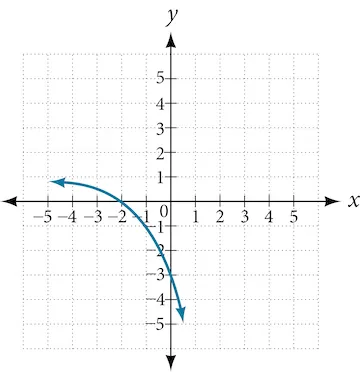39.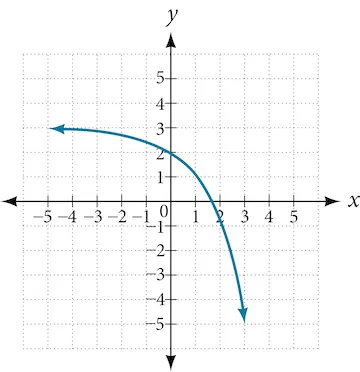40.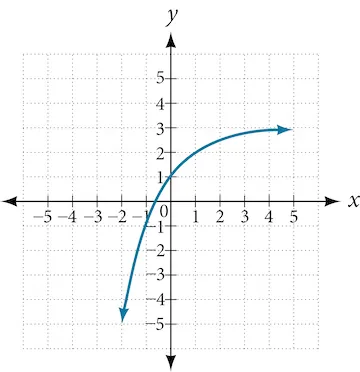For the following exercises, find an exponential equation for the graph.

41.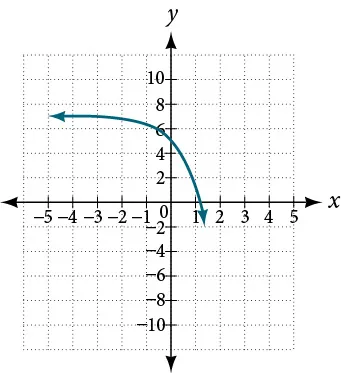42.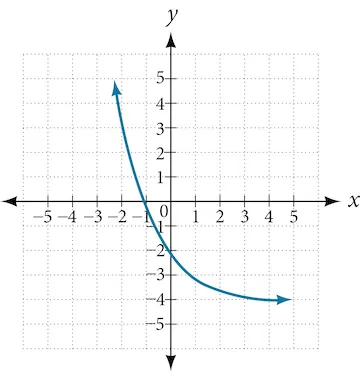#### Numeric

For the following exercises, evaluate the exponential functions for the indicated value of $x. x.$

43.

$g(x)= 1 3 ( 7 ) x−2 g(x)= 1 3 ( 7 ) x−2$ for $g(6). g(6).$

44.

$f(x)=4 (2) x−1 −2 f(x)=4 (2) x−1 −2$ for $f(5). f(5).$

45.

$h(x)=− 1 2 ( 1 2 ) x +6 h(x)=− 1 2 ( 1 2 ) x +6$ for $h(−7). h(−7).$

#### Technology

For the following exercises, use a graphing calculator to approximate the solutions of the equation. Round to the nearest thousandth.

46.

$−50=− ( 1 2 ) −x −50=− ( 1 2 ) −x$

47.

$116= 1 4 ( 1 8 ) x 116= 1 4 ( 1 8 ) x$

48.

$12=2 ( 3 ) x +1 12=2 ( 3 ) x +1$

49.

$5=3 ( 1 2 ) x−1 −2 5=3 ( 1 2 ) x−1 −2$

50.

$−30=−4 ( 2 ) x+2 +2 −30=−4 ( 2 ) x+2 +2$

#### Extensions

51.

Explore and discuss the graphs of $F(x)= ( b ) x F(x)= ( b ) x$ and $G(x)= ( 1 b ) x . G(x)= ( 1 b ) x .$ Then make a conjecture about the relationship between the graphs of the functions $b x b x$ and $( 1 b ) x ( 1 b ) x$ for any real number $b>0. b>0.$

52.

Prove the conjecture made in the previous exercise.

53.

Explore and discuss the graphs of $f(x)= 4 x , f(x)= 4 x ,$ $g(x)= 4 x−2 , g(x)= 4 x−2 ,$ and $h(x)=( 1 16 ) 4 x . h(x)=( 1 16 ) 4 x .$ Then make a conjecture about the relationship between the graphs of the functions $b x b x$ and $( 1 b n ) b x ( 1 b n ) b x$ for any real number n and real number $b>0. b>0.$

54.

Prove the conjecture made in the previous exercise.